Geo - activity 1-2 Practice wkst
1 point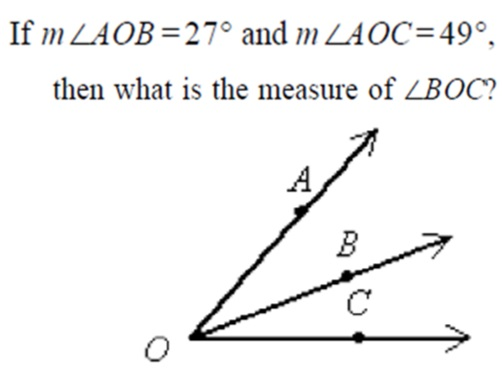Period *
Supplementary angles are.... *
1 point
Identify the wrong way to name this angle *
1 point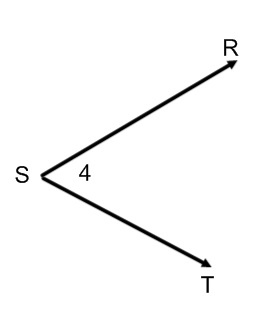What is measure of angle 1? *
1 point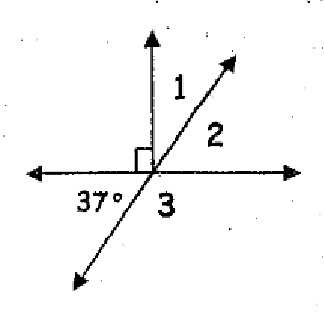Solve *
1 point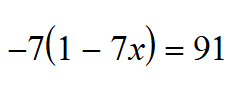Combine like terms and simplify *
1 point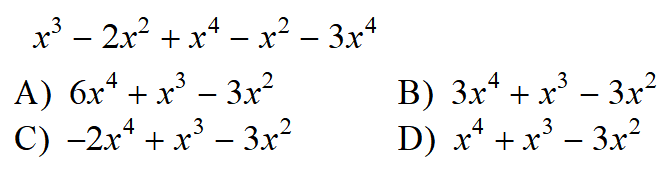What is measure of angle 3? *
1 pointLast name *
These angles are.... *
1 point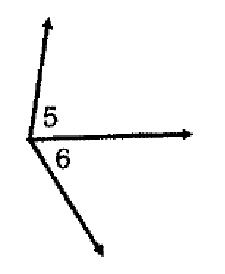What is measure of angle 2? *
1 point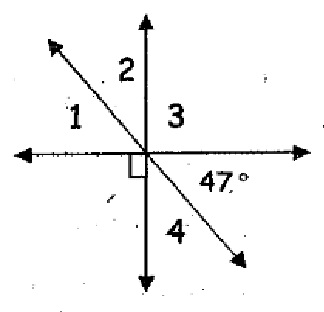What is measure of angle 4? *
1 point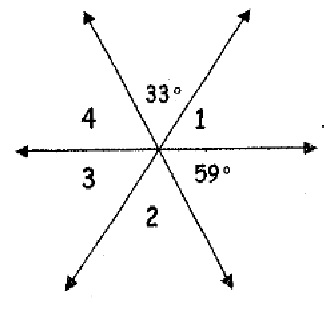Solve *
1 point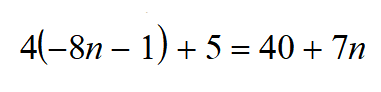sub in values and evaluate *
1 point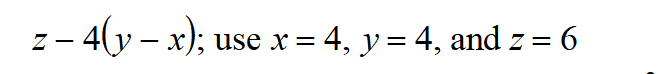Find x. *
1 point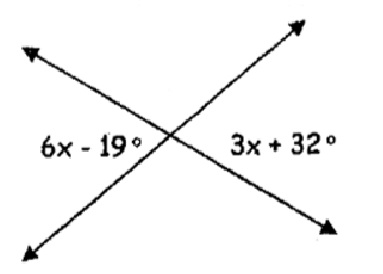Find x. *
1 point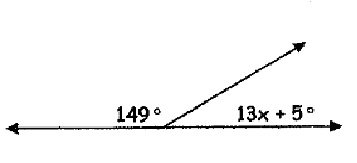Find x. *
1 point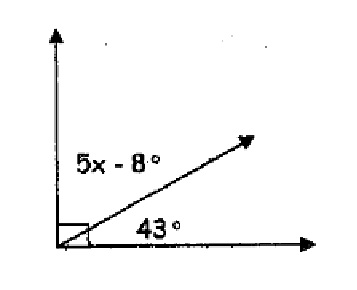Which is False? *
1 point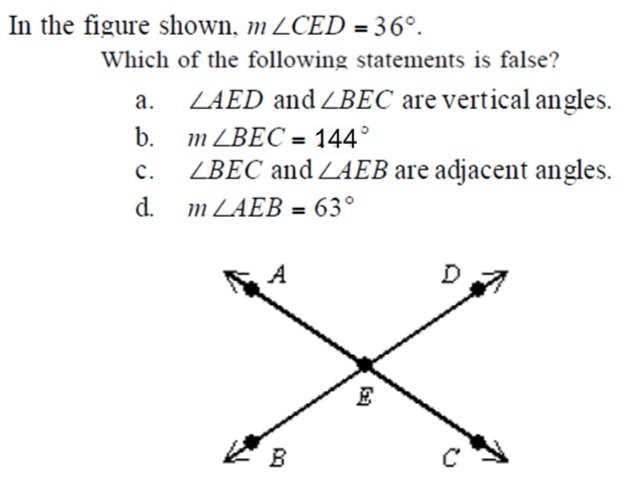period *
What is the measure of angle 3? *
1 pointWhat is measure of angle 3? *
1 pointFind k *
1 point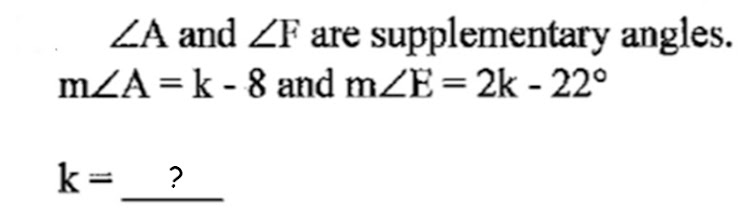Angle A and X are complementary.. If angle X is 33 degrees, What is measure of angle A? *
1 point